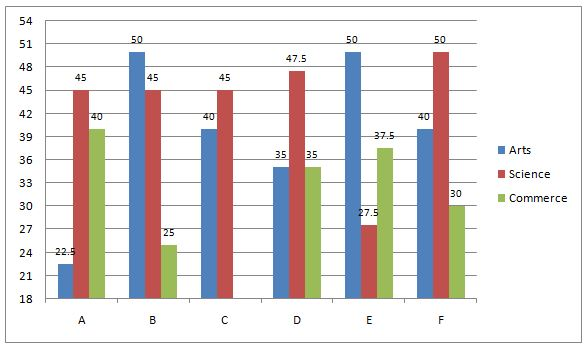## RBI Assistant Quant Test 12

Instructions

Study the following Graph carefully and answer the questions given below:Q 1

The number of students taking Arts in college B, is how many thousand fewer than the number of students taking Arts in college A and college C together ?

Q 2

In college F, the students taking Commerce is what percent of the total number of students taking Arts, Science and Commerce in all ?

Q 3

How many candidates (in thousand) have taken Commerce from all the colleges ?

Q 4

What is the respective ratio of the number of the students taking Science to the number of Students taking Arts in college D ?

Q 5

What is the average number of students (in thousand) taking up Science from all the six colleges together? (Rounded off to two digits after decimal)